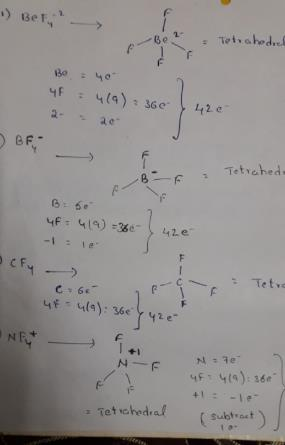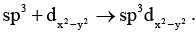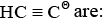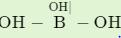Courses

# Test: Chemical Bonding- 2

## 30 Questions MCQ Test Inorganic Chemistry | Test: Chemical Bonding- 2

Description
This mock test of Test: Chemical Bonding- 2 for Chemistry helps you for every Chemistry entrance exam. This contains 30 Multiple Choice Questions for Chemistry Test: Chemical Bonding- 2 (mcq) to study with solutions a complete question bank. The solved questions answers in this Test: Chemical Bonding- 2 quiz give you a good mix of easy questions and tough questions. Chemistry students definitely take this Test: Chemical Bonding- 2 exercise for a better result in the exam. You can find other Test: Chemical Bonding- 2 extra questions, long questions & short questions for Chemistry on EduRev as well by searching above.
QUESTION: 1

Solution:
QUESTION: 2

Solution:
QUESTION: 3

### Which of the following set of ions/ molecules is isoelectric and structural?

Solution:Isoelectronic species are those have same number of electrons.

QUESTION: 4

In allene (C3H4), the type(s) of hybridisation of the carbon atoms is (are)

Solution:
QUESTION: 5

The atom that form discrete polyatomic molecule in its elemental state are:

Solution:

Sulfur forms discrete polyatomic molecule as in S8

Carbon forms discrete polyatomic molecules as in diamond, etc.

QUESTION: 6

Which one of the following pairs is isoelectronic?

Solution:
QUESTION: 7

The structure of XeF4 is:

Solution:
QUESTION: 8

Which of the following species has two non bonded electron pairs on the central atom:

Solution:

In TeCl4 out of 6 electrons:bonded electrons are 4,non-bonded electron pair is 1.
In ClF3 out of 7 electrons:bonded electrons are 3,non-bonded electron pairs are 2.
In PCl3 out of 5 electrons:bonded electrons are 3,non-bonded electron pair is 1.
In ICl2− out of (7+1=8) electrons:bonded electrons are 2,non-bonded electron pairs are 3.

QUESTION: 9

The shape and expected hybridization of BrO3- and HOCl are:

Solution:
QUESTION: 10

I3- ion is linear having the hybridization:

Solution:
QUESTION: 11

The hybridization of atomic orbital of nitrogen in NO2+, NO3- and NH4+ are:

Solution:
QUESTION: 12

Among the molecular ions, the dπ - pπ bond is possible in:

Solution:
QUESTION: 13Now the shape becomes:

Solution:
QUESTION: 14

Angles in XeO3F2 are equal with which molecule:

Solution:
QUESTION: 15

Covalent-molecules are usually held in a crystal structure by

Solution:

The atoms in molecular covalent molecules are held together by strong covalent bonds. Although these bonds are strong, there are only weak forces of attraction between molecules. These weak attractive forces are called van der Waal’s forces and can be broken with little energy.

QUESTION: 16

Hybridization on anionic carbon in allyl anion is:

Solution:
QUESTION: 17

Hybridization and shape of N(SiH3)3 is:

Solution:
QUESTION: 18

Hybridization in carbon atoms ofSolution:
QUESTION: 19

Hybridization in (C6H5)3C (on negative carbon) is:

Solution:
QUESTION: 20

Hybridization of carbon C3O2 and CO2 are:

Solution:
QUESTION: 21

Hybridization on central atom in SOF4 is:

Solution:
QUESTION: 22

Hybridization on central atom in Be2C is:

Solution:
QUESTION: 23

Hybridization in N2 is:

Solution:

Pi bond electrons don’t contribute when we calculate the hybridization. So due to one sigma bond and one lone pair hybridization will be “sp”.

Hence D is correct.

QUESTION: 24

Hybridization on the central atom in H3Ois:

Solution:
QUESTION: 25

Hybridization in Si in Si4O116– is:

Solution:
QUESTION: 26

In octahedral structure the pair of ‘d’ orbitals invo lved is:

Solution:
QUESTION: 27

The ONO angle is maximum is:

Solution:
QUESTION: 28

Hybridization state of boron and oxygen in boric acid is:

Solution:

The state of hybridization of boron and oxygen atom in boric acid (H3​BO3​) is sp2,sp3  respectively.

Boric acid; has a network structure in which boron is trigonal having sp2 and each oxygen atom is tetrahedral having sp3-hybridization with two lone pair of electrons on oxygen.

QUESTION: 29

Which is correct order of bond angle:

Solution:
QUESTION: 30

Which of These is Pyramidal in Shape?

Solution:

PCl3 has sp3-hybridised phosphorus, with one lone pair. Therefore, molecule has pyramidal shape like ammonia.